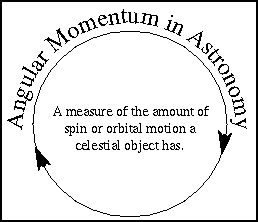# Angular Momentum

#### Chapter index in this window —   — Chapter index in separate window

This material (including images) is copyrighted!. See my copyright notice for fair use practices.## Definition

To describe how things move we often use the basic quantities of length, mass, and time. Quantities such as velocity, acceleration, force and energy are very powerful ones that help us understand how an object's position will change over time and how it will interact with other things in the universe. Momentum and its cousin angular momentum are other very powerful quantities.

Ordinary momentum is a measure of an object's tendency to move at constant speed along a straight path. Momentum depends on speed and mass. A train moving at 20 mph has more momentum than a bicyclist moving at the same speed. A car colliding at 5 mph does not cause as much damage as that same car colliding at 60 mph. For things moving in straight lines momentum is simply mass × speed. In astronomy most things move in curved paths so we generalize the idea of momentum and have angular momentum. Angular momentum measures an object's tendency to continue to spin. An ``object'' can be either a single body or two or more bodies acting together as a single group.

#### angular momentum = mass × velocity × distance (from point object is spinning or orbiting around)

Very often in astronomy, the object (or group of objects) we're observing has no outside forces acting on it in a way to produce torques that would disturb the angular motion of the object (or group of objects). A torque is simply a force acting along a line that is off the object's spin axis. In these cases, we have conservation of angular momentum.

#### conservation of angular momentum---the total amount of angular momentum does not change with time no matter how the objects interact with one another.

A planet's velocity and distance from the Sun will change but the combination of speed×distance will not change unless another planet or star passes close by and provides an extra gravity force.

#### Go to Astronomy Notes home

last updated: 27 May 2001